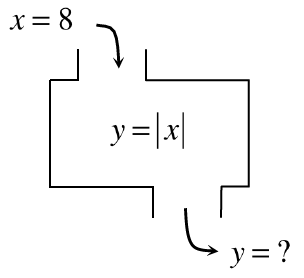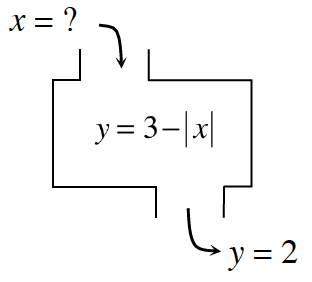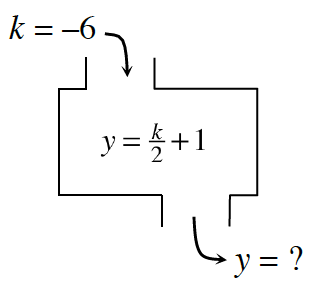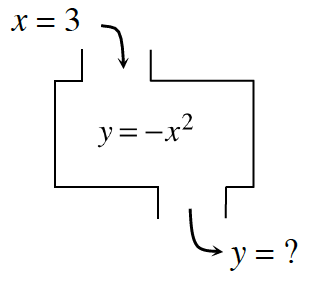### Home > CC3MN > Chapter 8 > Lesson 8.3.1 > Problem8-124

8-124.

Find the corresponding inputs or outputs for the following relationships. If there is no solution, explain why not. Be careful: In some cases, there may be no solution or more than one possible solution.

1.What is the absolute value of $8$?

1.Solve the equation by inspection.

$x = ±1$

1.Substitute and evaluate.

1.What is $3$ squared?

$−9$